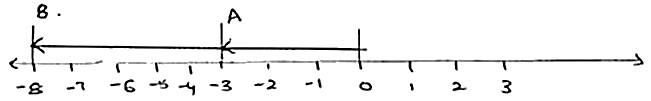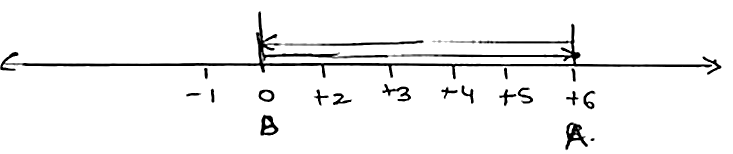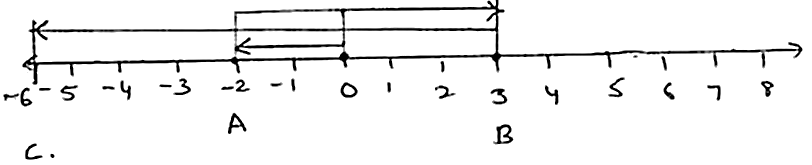×#### Thank you for registering.

One of our academic counsellors will contact you within 1 working day.

Click to Chat

1800-1023-196

+91-120-4616500

CART 0

• 0

MY CART (5)

Use Coupon: CART20 and get 20% off on all online Study Material

ITEM
DETAILS
MRP
DISCOUNT
FINAL PRICE
Total Price: Rs.

There are no items in this cart.
Continue Shopping• Complete JEE Main/Advanced Course and Test Series
• OFFERED PRICE: Rs. 15,900
• View Details

```Chapter 5: Negative Numbers and Integers – Exercise 5.2

Negative Numbers and Integers – Exercise – 5.2 – Q.1

Ans.

(i) 5 + (-2)We begin at 0 and first more five units to the right of zero to reach ar a which represents +5. The second number 2 is negative. So, we move 2 units to the left of A to reach at B which represents 3.

Thus, we have = 5 + (-2) = 3.

(ii) (-9) + 4.We begin at 0 and first move nine units to the left of zero to reach at A. Which represents – 9. The second number 4 is positive. So we over 4 units to the right of A to reach at B. Which represents -5.

Thus, we have.

-9 + 4 = -5.

(iii) (-3) + (-5).We begin at 0 and first move three units to the left of zero to reach at A which represents -3. The second number +5 is negative. So we move 5 units to the left of A to reach at b which represents -8.

Thus we have = (-3) + (-5) = -3 -5 = -8.

(iv) (-1) + (-2) + 2.We begin at zero and first move one unit to the left of zero to reach at point A which represents -1. The second number 2 is negative.

So we move 2 units to the left of A to reach at B which represents -3. The Third number is 2 positive. So we move 2 units to the right of B. which is -1.

(-1) + (-2) + 2 = (-1) + 2 -2 = -1.

(v) 6 + (-6)Thus we have 6 + (-6) = 0

(vi) (-2) + 5 + (-a).Thus we have = -2 + 5 + (-9)

= 5 - 2 + (-9)

= 3 - 9

= -6.

Negative Numbers and Integers – Exercise – 5.2 – Q.2

Ans.

(i) – 557 and 488

The integers are to be added are of the unlike signs.Therefore to add them we find the difference of their absolute values and assign the sign of the addend having greater absolute value

(- 557) and 488 = |- 557| - |488|

= 557 - 488

= - 69.

(ii) - 522 + (-160) = - 522 - 160

= - 682

(iii) 2567 and -325

2567 + (-325) = (2567) - (-325)

= 2567 and -325

2567 + (-325) = (2567) - (-325)

= 2567 - 325

= 2242

(iv) – 10025 and 139

-10025 + 139 = [-10025] + 

= -10025 + 139

= -9886.

(v) 2567+ (-2578) = 2547 - 2548

= -1.

(vi) 2884 + (-2884) = 2884 - 2884 = 0.
```### Course Features

• 728 Video Lectures
• Revision Notes
• Previous Year Papers
• Mind Map
• Study Planner
• NCERT Solutions
• Discussion Forum
• Test paper with Video Solution